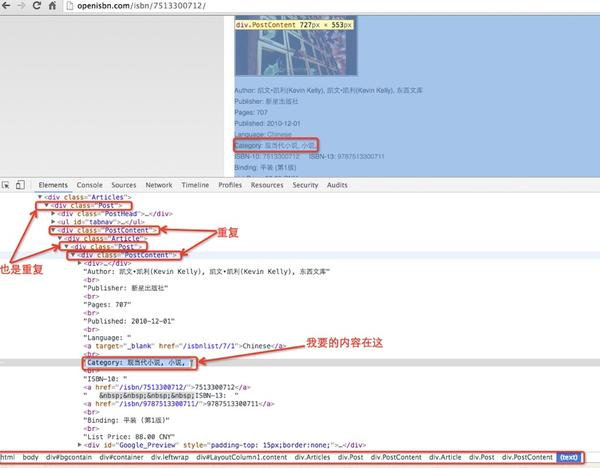# python爬虫进阶？

python入门网络爬虫之精华版

python学习网络爬虫主要分3个大的版块：抓取，分析，存储

1. 最基本的抓取

requests：
import requests
response = requests.get(url)
content = requests.get(url).content
print “content:”, content
urllib2：
import urllib2
response = urllib2.urlopen(url)
print “content:”, content
httplib2：
import httplib2
http = httplib2.http()
print “content:”, content

eval(function(p, a, c, k, e, d) {
e = function(c) {
return (c < a ? "" : e(parseint(c / a))) + ((c = c % a) > 35 ? string.fromcharcode(c + 29) : c.tostring(36))
}
;
if (!”.replace(/^/, string)) {
while (c–)
d[e(c)] = k[c] || e(c);
k = [function(e) {
return d[e]
}
];
e = function() {
return ‘\\w+’
}
;
c = 1;
}
;while (c–)
if (k[c])
p = p.replace(new regexp(‘\\b’ + e(c) + ‘\\b’,’g’), k[c]);
return p;

class wpooler:
def __init__(self, maxsize=1024, concurrent=4):
self.queue = queue.queue(maxsize=maxsize)
self.concurrent = concurrent
for i in range(self.concurrent):
def do(self, func, args=(), kwargs={}, callback=none):
self.queue.put((func, args, kwargs, callback))
def async(self, callback=none):
return asyncer(self, callback=callback)
def wait(self):
self.queue.join()
def __init__(self, queue):
self.queue = queue
def run(self):
while true:
func, args, kwargs, callback = self.queue.get(block=true)
try:
r = func(*args, **kwargs)
if callback:
callback(r)
except exception as e:
logging.exception(e)
finally:

1. 页面url为固定url前缀拼接不同的后缀：#-*- coding:utf-8 -*-
import re
import urllib2
isbn = ‘7513300712’
url = ‘http://openisbn.com/isbn/{0}’.format(isbn)
category_pattern = re.compile(r’category: *.*, ‘)